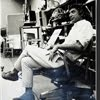## Eugene Ray SDSU 1970'sEugene Ray 1970's. photo credit: Tom Davis

## Friday, January 25, 2013

### PRECIOUS DAYS on this PLANET posterity shall decide our place ! ^^^^^^^^^^^^^^^^^^^^^ LIFE HAS MANY DIMENSIONS & for me >louisiana & california<- ZONES to LEARN=GROW=BUILD ^^^^^^^^^^^^^^^^^^^^^ GRATITUDE to ROBET GAUGHEN & ANN FLEMING old/new friends ^^^^^^^^^^^^^^^^^^^^^ 5 PHOTOS=CALIFORNIA OEUVRE #1) radiant secrets into the light #2) retrospective exhibition 1980 #3) radiant strength architecture #4) biotronic inflatable structure #5) sdsu classroom/studio 70-80 Inbox x+GRATITUDE+
>to new friend ann fleming who sent<
>me a national geographic link re:<
+JOAN OF ARC+
>who crossed the aube river with<
+KING RENE D'ANJOU+
>near the degruy chateau at<
+MAGNY FOUCHARD+
>approx six hundred years ago<
^^^^^^^^^^^^^^^

>URVILLE ON THE PILGRIMAGE+
+IS CLOSE TO CLAIRVAUX+
>st bernard abby<
+& MAGNY FOUCHARD+
^^^^^^^^^^^

+DEDICATION+
>tonights photos for robert gaughen<
^^^^^^^^^^^^^^^^^^^^
>who like dave fobes, tom davis<
>ralph bowman, mario lara<
>nancie greenfield<
>roger utt, john holland and<
>other early seventies<
+SDSU ALUMNI+
^^^^^^^^
>were w/ me in the bell tower<
+OLD LIBRARY+
>studio classroom lab where<
^^^^^^^^^^^

+TONIGHT'S PHOTOS+
>ten early years of my oeuvre<
>in california traces vital<
+1970-1980+
>work development<
^^^^^^^^^^

>into the light<
^^^^^^
+L U C E S+
>exhibition in the last full year<
+1 9 9 5+
>before my retirement of the<
>work w/ color, light and<
+E N E R G Y+
^^^^^^
>bob wil remember the sdsu class<
+SYNERGETIC ENVIRONS+
>was based on this radiant work<
^^^^^^^^^^^^^^^^^^

#2)          +DOCUMENTA 1980+
>was an exhibition of the california<
>oeuvre, the first ten years, which<
>culminated w/ the "silver ship"<
^^^^^^^^^^^^^^^^
+D O M U S+
>publcation the next year and the<
+PARIS BIENNAL 1985+
>and<
+A+U TOKYO 1985+
^^^^^^^^^^^^^^^

#3)        +SPHERICAL VOLUME+
>with biotronic energy, gardens and<
>extra strong enclosure defying<
+STORMS & QUAKES+
>became our prototypes focus<
^^^^^^^^^^^^^^^
+ROBERT GAUGHEN+
>will remember my effort to open<
>the outer shell w/ the design<
+UNIVERSAL SPHEROID+
^^^^^^^^^^^^

#4)           +INFLATABLES+
>in the seventies on we built a series<
>of inflatables for exhibitions and<
+SYNERGETIC ENVIRONS+
^^^^^^^^^^^^^
>bob gaughen will remember our<
>camping trip to arcosanti and<
>taliesin west using a large<
+AIR STRUCTURE+
(>cost fifty dollars housing<
>fifty sdsu/ed students<)
^^^^^^^^^^

>galore filled our seventies sdsu/ed<
>studio/classroom where tensile<
>biomorphic structures with<
+BIOTRONIC+
^^^^^^^^^^^^^^^^^
+ROBERT GAUGHEN+
>and many others were part of<
>that history that this blog<
>nightly tries to illuminate<
+SYNCHRONISTICALLY+
^^^^^^^^^^^

+ROBERT BIGELOW+
>as an example was building his<
>las vegas based hotel empire<
^^^^^^^^^^^^^^^^
+AND DEEP INTO UFO+
>extraterrestrial<
+RESEARCH THAT WOULD<
>bigelow aerospace<
+IGNITE HIS SPACE EFFORT+
^^^^^^^^^^^^^^
>nasa just awarded him a special<
>eighteen million dollar contract<
>to build his space station<
+INFLATABLE+
^^^^^^

+POIGNANTLY+
>last night i watched the interview<
>w/ robert lazar before he was<
+MURDERED+
^^^^^^^^^^^^^^^^
+ARE DOUBLE LOCKED+
^^^^^^^^^^
>remember professor john mack<
>harvard abduction specialist<
+RUN OVER IN LONDON+
^^^^^^^^^^^^^
>look for "the man who<
+GOT AWAY+
^^^^^^

gene ray, lajolla, california

>eugene ray, mfa, architect<
>professor emeritus, sdsu<
^^^^^^^^^^^^^^